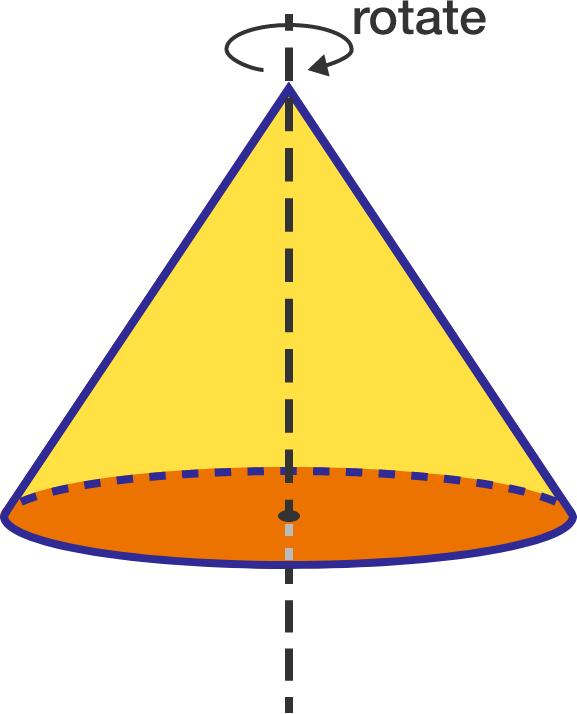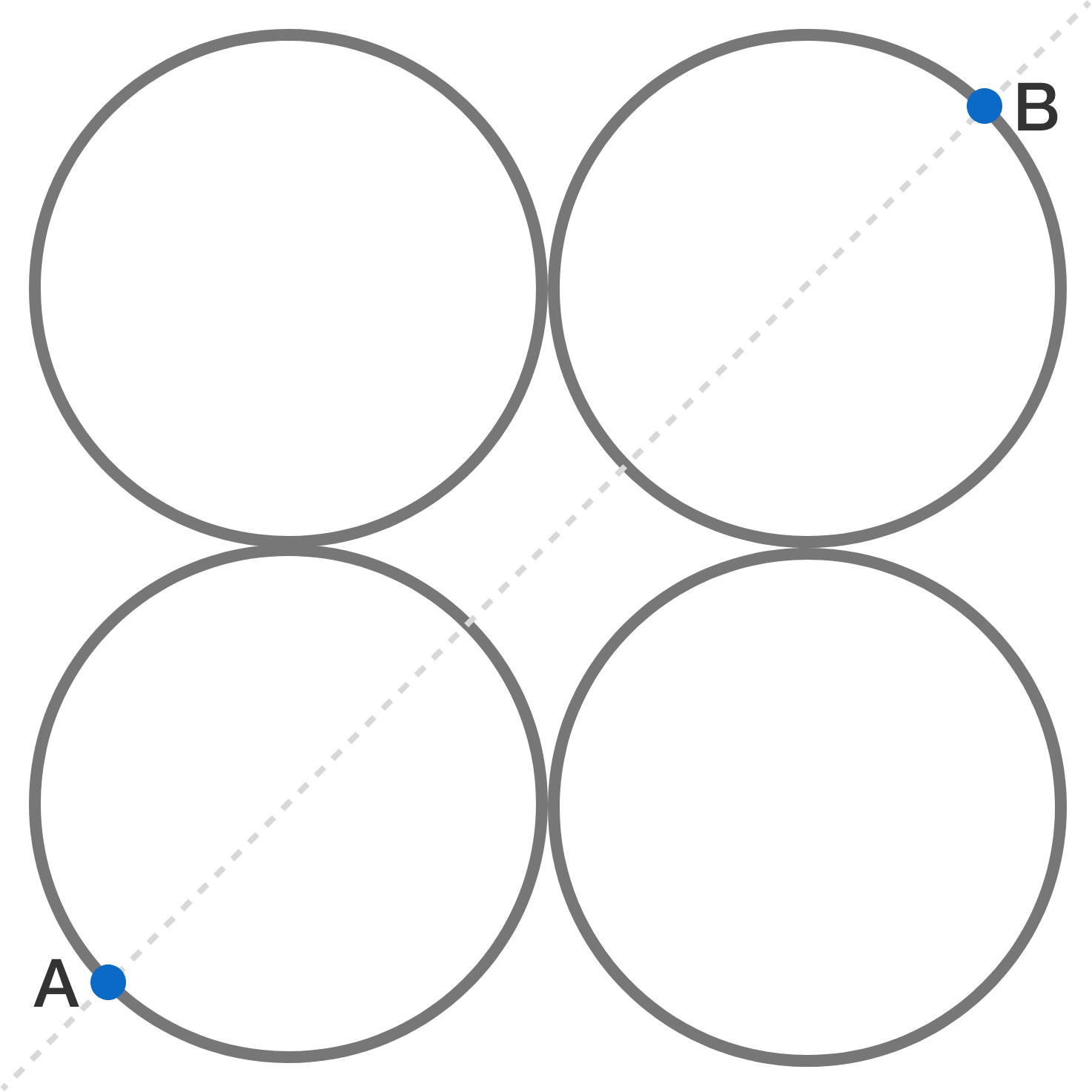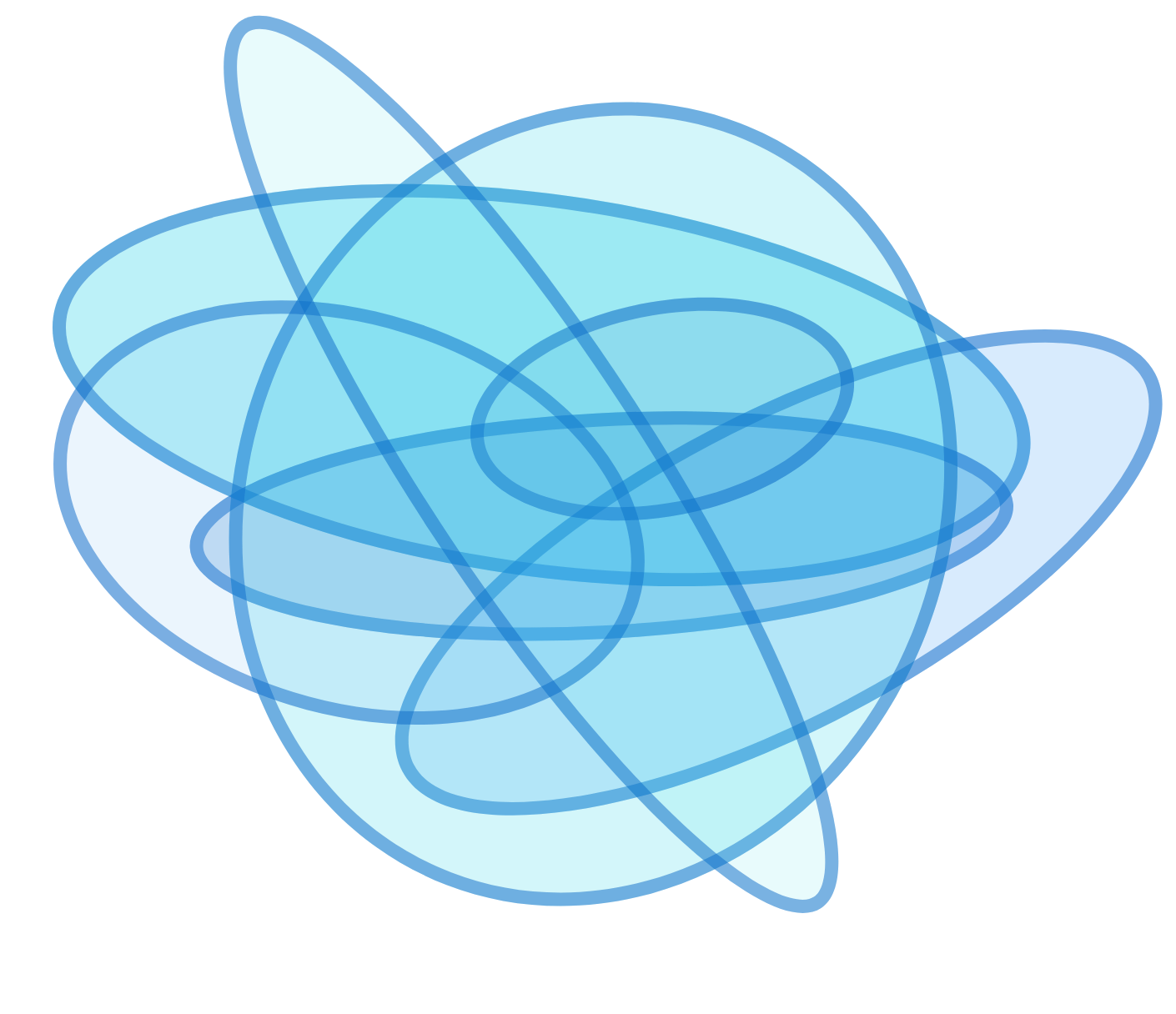# Problems of the Week

Contribute a problemA uniform solid right-circular cone of mass $M$ and radius $R$ is kept on a rough horizontal floor (coefficient of friction $\mu$) on its circular base. It is spun with initial angular velocity $\omega_{0}$ about its symmetry axis. Neglecting toppling effects (if any), find the time after which it stops spinning.


Details and Assumptions:

• Take the moment of inertia of the cone about its symmetry axis as $I$.

###### If you are looking for more such twisted questions, Twisted problems for JEE aspirants is for you!

$x,y,z,t$ are all integers satisfying the following system of equations: \begin{cases} \begin{aligned} xz-2yt&=3 \\ xt+yz&=1. \end{aligned} \end{cases} Find $x^2+y^2+z^2+ t^2$.A uniform wire of resistance $R$ is cut into four circular rings of equal radius. Rings are then connected such that their centers lie on the vertices of a square (as illustrated in the figure above).

If the equivalent resistance between $A$ and $B$ can be expressed as $\frac{aR}{b}$, where $a$ and $b$ are coprime positive integers, determine the value of $a+b$.

$S= \frac{1^{2}}{10}+\frac{2 \times 1^{2}+2^{2}}{10^{2}}+\frac{3 \times 1^{2}+2 \times 2^{2}+3^{2}}{10^{3}}+\cdots$

The sum $S$ defined above is an infinite sum whose $n^\text{th}$ term is $\dfrac{n \times 1^{2}+(n-1) \times 2^{2}+(n-2) \times 3^{2} + \cdots + n^{2}}{10^{n}}$ for $n=1,2,\ldots$.

If $S$ can be expressed in the form $\frac{a}{b}$, where $a$ and $b$ are coprime positive integers, find $a+b$.What is the maximum number of regions a plane may be cut into by 2017 ellipses?

×